# 0. 社区划分简介

## 0x1：非重叠社区划分方法

### 1. 基于模块度优化的社区划分

1. 凝聚方法(down to top): 通过不断合并不同社区，实现对整个网络的社区划分，典型的方法有Newman快速算法，CNM算法和MSG-MV算法；
2. 分裂方法(top to down): 通过不断的删除网络的边来实现对整个网络的社区划分，典型的方法有GN算法；
3. 直接近似求解模块度函数(近似等价解): 通过优化算法直接对模块度函数进行求解，典型的方法有EO算法；

### 2. 基于谱分析的社区划分算法3. 基于信息论的社区划分算法4. 基于标签传播的社区划分算法

undone

https://www.cnblogs.com/LittleHann/p/9078909.html

# 1. Label Propagation简介

LPA是一种基于标签传播的局部社区划分。对于网络中的每一个节点，在初始阶段，Label Propagation算法对于每一个节点都会初始化一个唯一的一个标签。每一次迭代都会根据与自己相连的节点所属的标签改变自己的标签，更改的原则是选择与其相连的节点中所属标签最多的社区标签为自己的社区标签，这就是标签传播的含义了。随着社区标签不断传播。最终，连接紧密的节点将有共同的标签。

## 0x1：LPA基本思想

LPA认为每个结点的标签应该和其大多数邻居的标签相同，将一个节点的邻居节点的标签中数量最多的标签作为该节点自身的标签（bagging思想）。给每个节点添加标签（label）以代表它所属的社区，并通过标签的“传播”形成同一个“社区”内部拥有同一个“标签”。

1. 首先: Kmeans是基于欧式空间计算节点向量间的距离的，而LPA则是根据节点间的“共有关系”以及“共有关系的强弱程度”来度量度量节点间的距离；
2. 第二点: Kmeasn中节点处在欧式空间中，它假设所有节点之间都存在“一定的关系”，不同的距离体现了关系的强弱。但是 LPA 中节点间只有满足“某种共有关系”时，才存在节点间的边，没有共有关系的节点是完全隔断的，计算邻居节点的时候也不会计算整个图结构，而是仅仅计算和该节点有边连接的节点，从这个角度看，LPA 的这个图结构具有更强的社区型；

## 0x2：LPA算法优点

LPA算法的最大的优点就是算法的逻辑非常简单，相对于优化模块度算法的过程是非常快的，不用pylouvain那样的多次迭代优化过程。
LPA算法利用自身的网络的结构指导标签传播，这个过程是无需任何的任何的优化函数，而且算法初始化之前是不需要知道社区的个数的，随着算法迭代最后可以自己知道最终有多少个社区。

## 0x3：LPA算法缺点

1. 更新顺序：节点标签更新顺序随机，但是很明显，越重要的节点越早更新会加速收敛过程；
2. 随机选择：如果一个节点的出现次数最大的邻居标签不止一个时，随机选择一个标签作为自己标签。这种随机性可能会带来一个雪崩效应，即刚开始一个小小的聚类错误会不断被放大。不过话也说话来，如果相似邻居节点出现多个，可能是weight计算的逻辑有问题，需要回过头去优化weight抽象和计算逻辑；

## 0x4：LPA的一个简单例子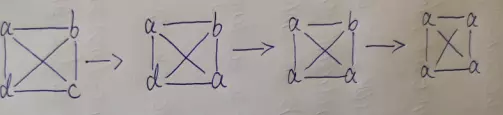d也根据自己周围的邻居节点来确定标签数，最多的是a，所以就是d为a了；

https://www.jianshu.com/p/cff65d7595f9
https://arxiv.org/pdf/0709.2938.pdf
https://blog.csdn.net/Katherine_hsr/article/details/82343647
http://sighingnow.github.io/%E7%A4%BE%E4%BC%9A%E7%BD%91%E7%BB%9C/community_detection_k_means_clustering.html

# 2. LPA算法过程

## 0x2：边权重计算

1. 两个DNS域名共享的client ip数量；
2. 两个微博ID的共同好友数量；

## 0x3：标签传播方式

LPA标签传播分为两种传播方式，同步更新，异步更新。

### 1. 同步更新，其中表示的就是节点在第 t 次迭代时的社区标签。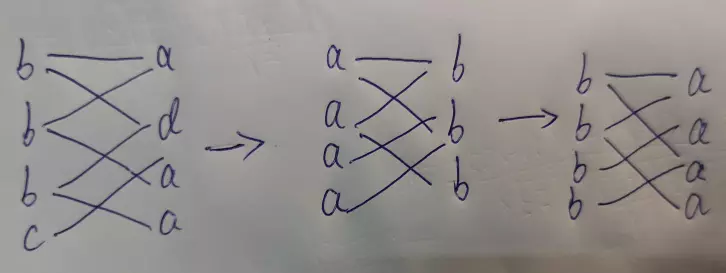## 0x4： 算法代码

### 1. 数据集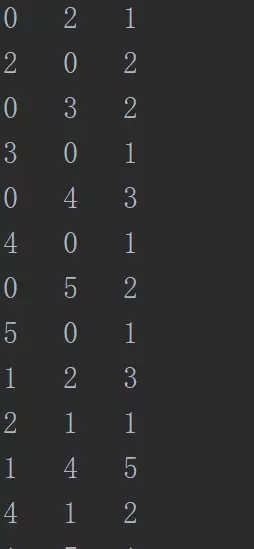3列分别是：【node_out，node_in，edge_weitght】

### 2. 社区初始化

import matplotlib.pyplot as plt
import pandas as pd
import numpy as np
import string

f = open(filePath)
vector_dict = {}
edge_dict = {}
lines = line.strip().split("   ")
for i in range(2):
if lines[i] not in vector_dict:
vector_dict[lines[i]] = int(lines[i])
edge_list = []
if len(lines) == 3:
edge_list.append(lines[1 - i] + ":" + lines)
else:
edge_list.append(lines[1 - i] + ":" + "1")
edge_dict[lines[i]] = edge_list
else:
edge_list = edge_dict[lines[i]]
if len(lines) == 3:
edge_list.append(lines[1 - i] + ":" + lines)
else:
edge_list.append(lines[1 - i] + ":" + "1")
edge_dict[lines[i]] = edge_list
return vector_dict, edge_dict

if __name__ == '__main__':
filePath = './label_data.txt'
print(vector)
print(edge)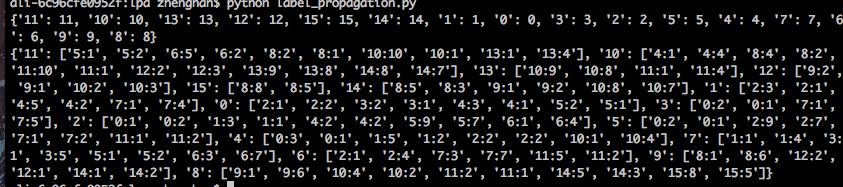### 3. LPA社区聚类迭代

# -*- coding: utf-8 -*-
import matplotlib.pyplot as plt
import pandas as pd
import numpy as np
import string

f = open(filePath)
vector_dict = {}
edge_dict = {}
lines = line.strip().split("   ")
for i in range(2):
if lines[i] not in vector_dict:
vector_dict[lines[i]] = int(lines[i])
edge_list = []
if len(lines) == 3:
edge_list.append(lines[1 - i] + ":" + lines)
else:
edge_list.append(lines[1 - i] + ":" + "1")
edge_dict[lines[i]] = edge_list
else:
edge_list = edge_dict[lines[i]]
if len(lines) == 3:
edge_list.append(lines[1 - i] + ":" + lines)
else:
edge_list.append(lines[1 - i] + ":" + "1")
edge_dict[lines[i]] = edge_list
return vector_dict, edge_dict

label_dict = {}
node_id_weight = node.strip().split(":")
node_id = node_id_weight
node_weight = int(node_id_weight)

# 按照label为group维度，统计每个label的weight累加和
if vector_dict[node_id] not in label_dict:
label_dict[vector_dict[node_id]] = node_weight
else:
label_dict[vector_dict[node_id]] += node_weight

sort_list = sorted(label_dict.items(), key=lambda d: d, reverse=True)
return sort_list

def check(vector_dict, edge_dict):
for node in vector_dict.keys():
node_label = vector_dict[node]          # 获取该节点当前label
label = get_max_community_label(vector_dict, adjacency_node_list)   # 从邻居节点列表中选择weight累加和最大的label
if node_label >= label:
continue
else:
return 0    #  找到weight权重累加和更大的label
return 1

def label_propagation(vector_dict, edge_dict):
t = 0
print('First Label: ')
while True:
if (check(vector_dict, edge_dict) == 0):
t = t + 1
print('iteration: ', t)
# 每轮迭代都更新一遍所有节点的社区label
for node in vector_dict.keys():
else:
break
return vector_dict

if __name__ == '__main__':
filePath = './label_data.txt'
print "load and initial the community...."
#print(vector)
#print(edge)
print "start lpa clustering...."
vector_dict = label_propagation(vector, edge)
print "ending lpa clustering...."
print "the finnal cluster result...."
print(vector_dict)

cluster_group = dict()
for node in vector_dict.keys():
cluster_id = vector_dict[node]
print "cluster_id, node", cluster_id, node
if cluster_id not in cluster_group.keys():
cluster_group[cluster_id] = [node]
else:
cluster_group[cluster_id].append(node)

print cluster_group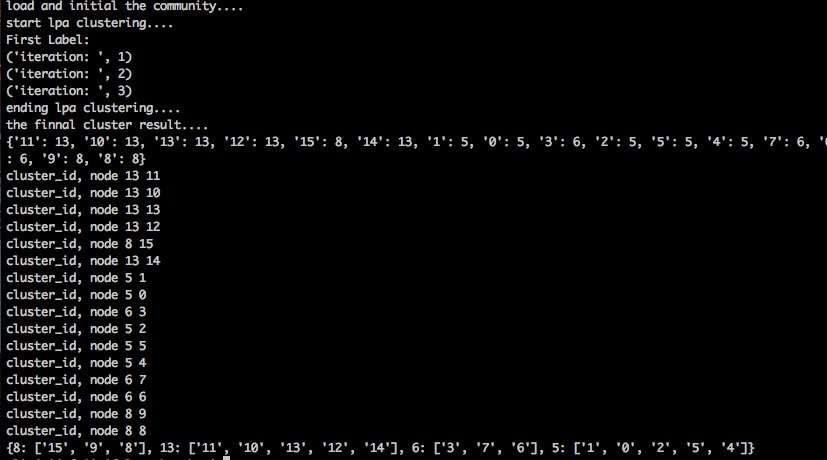{8: ['15', '9', '8'], 13: ['11', '10', '13', '12', '14'], 6: ['3', '7', '6'], 5: ['1', '0', '2', '5', '4']}

https://github.com/GreenArrow2017/MachineLearning/tree/master/MachineLearning/Label%20Propagation
https://www.jianshu.com/p/cff65d7595f9

# 3. LPA算法改进思路

## 0x2：标签初始化改进

https://www.cnblogs.com/bethansy/p/6953625.html
https://blog.csdn.net/zzz24512653/article/details/26151669